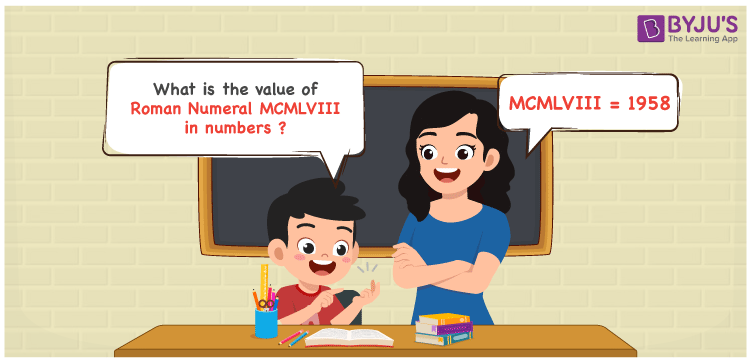Checkout JEE MAINS 2022 Question Paper Analysis : Checkout JEE MAINS 2022 Question Paper Analysis :

# MCMLVIII Roman Numerals

MCMLVIII Roman Numerals is 1958. Roman Numerals is the system of representing numbers using the Roman alphabet. Students can make use of Roman Numerals 1 to 1000  in PDF format for a thorough knowledge of how to express Roman letters from 1 to 1000 numbers. In this article, let us have a glance at how to convert MCMLVIII Roman Numeral into numbers accurately.

 Number Roman Numeral 1958 MCMLVIII

## How to Write MCMLVIII Roman Numerals?The following methods are used to denote the number form of MCMLVIII Roman Numerals

First Method:

• Break the Roman Numerals into single letters
• MCMLVIII = M + (M – C) + L + V + I + I + I
• Write the numerical value of each letter and add/subtract them
• MCMLVIII = 1000 + (1000 – 100) + 50 + 5 + 1 + 1 + 1 = 1958

Second Method:

In this method, we consider the groups of Roman Numerals for addition or subtraction

• MCMLVIII = M + CM + L + VIII = 1000 + 900 + 50 + 8 = 1958
• Therefore the numerical value of MCMLVIII Roman Numerals is 1958

## Video Lesson on Roman Numerals## Related Articles

Roman Numerals

Roman Numerals 1 to 50

Roman Numerals 1 to 100

Roman Numerals Conversion

L Roman Numerals

Roman Numeral XXXIX

## Frequently Asked Questions on MCMLVIII Roman Numerals

### What is MCMLVIII Roman Numerals?

MCMLVIII Roma Numerals is 1958.

### Find the value of (500 – 495) + 1958 in Roman Numerals.

(500 – 495) + 1958 = 1963. The number 1963 in Roman Numerals is MCMLXIII. Hence the value of (500 – 495) + 1958 in Roman Numerals is MCMLXIII.

### How do you write the number 1958 in Roman Numeral?

The number 1958 in Roman Numeral is written as MCMLVIII.